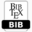## Mathematical Analysis of Tumour Invasion Model with Proliferation and Re-Establishment

 AUTHOR(S): Akisato Kubo TITLE Mathematical Analysis of Tumour Invasion Model with Proliferation and Re-EstablishmentPDFBIBTEX ABSTRACT We study the global existence in time and asymptotic profile of solutions of a mathematical model of tumour invasion proposed by Chaplain and Lolas. We consider related nonlinear evolution equations with strong dissipation, proliferation and an initial Neumann-boundary value problem. We show global existence in time of solutions to the initial boundary value problem in arbitrary space dimension by using the method of energy. Applying the result of existence and asymptotic behaviour of solutions to our problem we discuss the property of the solution to the tumour invasion model. Further we discuss a more general form of the nonlinear evolution equation, which could give the same type of existence theorem for a more general form of the tumour invasion model. KEYWORDS Nonlinear evolution equation, mathematical analysis, tumour invasion, cell proliferation, reestablishment of MDE REFERENCES  Anderson,A.R.A. and Chaplain, M.A.J., Continuos and discrete mathematical models of tumour-induced angiogenesis, Bull. Math. Biol., 60 (1998), 857–899.  Anderson,A.R.A. and Chaplain, M.A.J., Continuos and discrete mathematical models of tumour-induced angiogenesis, Bull. Math. Biol., 60 (1998), 857–899.  Anderson,A.R.A. and Chaplain, M.A.J., Mathematical modelling of tissue invasion, in Cancer Modelling and Simulation , ed. Luigi Preziosi, Chapman Hall/CRC (2003), .269-297.  Chaplain,M.A.J. and Lolas,G., Mathematical modeling of cancer of tissue: Dynamic heterogeneity, Networks and Heterogeneous Media, vol. 1,Issue 3 (2006), 399–439.  Dionne,P., Sur les problemes de Cauchy hyperboliques bien poses, J.Anal.Math., 10 (1962), 1– 90.  Kubo, A. and Kimura, K., Mathematical analysis of tumour invasion with proliferation model and simulations, WSEAS Transaction on Biology asnd Biomedicine, Vol.11, 2014, 165-173.  Kubo,A. and Suzuki,T., Asymptotic behavior of the solution to a parabolic ODE system modeling tumour growth, Differential and Integral Equations.,17(7-8) (2004), 721–736.  Kubo,A. and Suzuki,T. and Hoshino,H., Asymptotic behavior of the solution to a parabolic ODE system Mathematical Sciences and Applications.,22 (2005), 121-135.  Kubo,A. and Suzuki,T., Mathematical models of tumour angiogenesis, Journal of Computational and Applied Mathematics.,204 (2007), 48–55.  Kubo,A. Saito,N. Suzuki,T. and Hoshino,H., Mathematical models of tumour angiogenesis and simulations, Theory of Bio-Mathematics and Its Application., in RIMS Kokyuroku,1499 (2006), 135–146.  Kubo,A., Nonlinear evolution equations associated with mathematical models, Discrete and Continous Dynamical Systems, supplement 2011 (2011), 881–890.  Kubo, A., Mathematical Analysis of a model of Tumour Invasion and Simulations, International Journal of Mathematical Models and Methods in Applied Science, Issue 3, 4, 2010, 187-194.  Levine,H.A. and Sleeman,B.D., A system of reaction and diffusion equations arising in the theory of reinforced random walks, SIAM J. Appl. math.,57(3) (1997), 683–730.  Sleeman,B.D. and Levine,H.A., Partial differential equations chemotaxis and angiogenesis, Math. Mech. Appl. Sci., 24 (2001), 405–426.  Othmer,H.G. and Stevens,A., Aggregation, blowup, and collapse: The ABCs of taxis in reinforced random walks, SIAM J. Appl. ath.,57(4) (1997), 1044–1081.  Yang,Y. Chen,H. and Liu,W., On existence and non-existence of global solutions to a system of reaction-diffusion equations modeling chemotaxis, SIAM J. Math. Anal.,33 (1997), 763–785. Cite this paper Akisato Kubo. (2016) Mathematical Analysis of Tumour Invasion Model with Proliferation and Re-Establishment. International Journal of Mathematical and Computational Methods, 1, 115-119Copyright © 2016 Author(s) retain the copyright of this article.This article is published under the terms of the Creative Commons Attribution License 4.0